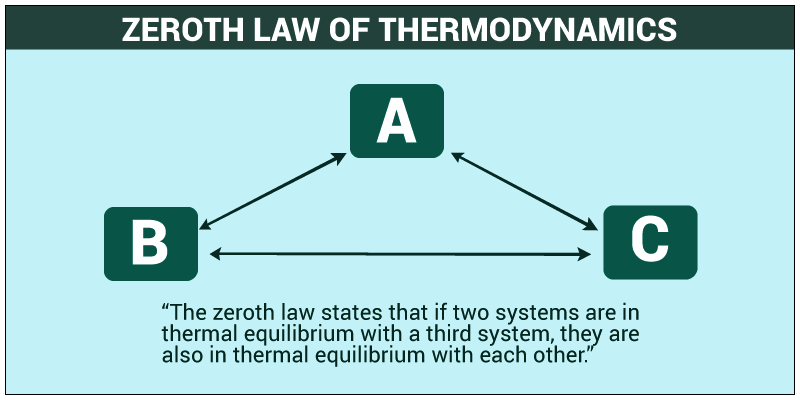# ALPHY6: THERMODYNAMICS

## Zeroth Law Of Thermodynamics:

The name Zeroth Law came in picture because all other three laws named First, Second and Third law were discovered earlier and the importance of Zeroth law was visualized as most important and hence can’t be given a number as Fourth Law. To mark the importance of the most basic law of thermodynamic the name Zeroth Law came into existence.When two systems are in thermal equilibrium with each other and any one of them separately or combined are in thermal equilibrium with a third system then they all arein thermal equilibrium with each other.

For eg:- If a body A is in thermal equilibrium with a body B & also separately with a body C then body B & C will be in thermal equilibrium with each other.Zeroth law of thermodynamics is basis of temperature measurement.

# 1st Law of Thermodynamics

Energy can be neither created nor destroyed (conservation of energy)

Thus power generation processes and energy sources actually involve conversion of energy from one form to another, rather than creation of energy from nothing

ΔQ = ΔU + ΔW

ΔU: Change in internal energy of the system

ΔQ: Heat transferred into/out of the system

ΔW: Work done by/on the systemCylinder has area, A. A fluid is admitted at constant pressure, p

p = F/A & Wd = fd … rearrange:

→ Wd = pV or ΔWd = pΔV

pV = nRT (Ideal Gas Law)

Boyle’s Law: pV = constant

• Temperature remains constant (isothermal)
• pV = constant and p1V1 = p2V2
• ΔU = 0 because the internal energy is dependent on temperature, which does not change
• ΔQ = ΔW. If the gas expands to do work ΔW, & amount of heat ΔQ must be supplied
• compression or expansion produces the same graph

Adiabatic: no heat flow (ΔQ=0) into or out of a system

For a change in pressure or volume in a system, the temperature loss can be calculated:

p1V1/T1 = p2V2/T2

At high p, low V: adiabatic = value expected for isothermal at high T

At low p, high V: adiabatic cuts isothermal at low TpVγ = k

γ = Cp/Cv

k= constant

Isovolumetric: p1T1 = p2T2Isobaric: V1T1 = V2T2# 2nd Law of Thermodynamics

2nd Law of Thermodynamics: Entropy of an isolated system not in equilibrium will tend to increase over time, approaching a maximum value at equilibrium – i.e. entropy increases & all processes tend towards chaos

Temperature gradient: Heat flows from a region of hot temperature to a region of cold temperature

All heat engines give up their energy to a cold reservoir

Qin:heat flow from the hot reservoir to the engine

Qout: heat flow from the engine to the cold reservoir.

Work done by heat engine = Qin – Qout

Efficiency = W/Qin = (Qin – Qout)/Qin

Limitations to Thermal Efficiency: – in an engine:

• TH cannot be too high > components could melt
• TC will be in the range of atmospheric temperatures
• Analysis of the engine cycle can help to improve efficiency
• Design of ports so that gas can get enter & exit with min. resistance
• Lubrication reduces friction in bearings

Therefore an engine will never work at its theoretical efficiency

THE FIRST AND SECOND LAWS OF THERMODYNAMICS

# The third law

Unlike the other laws, the third law is interpreted on the molecular level. It states that all processes in a system will cease to exist at a temperature of absolute zero, or alternatively no system can reach absolute zero. The molecules will stop moving at absolute zero, there will be no energy; this is why no system can reach absolute zero.

## STEM Elearning

We at FAWE have built this platform to aid learners, trainers and mentors get practical help with content, an interactive platform and tools to power their teaching and learning of STEM subjects, more